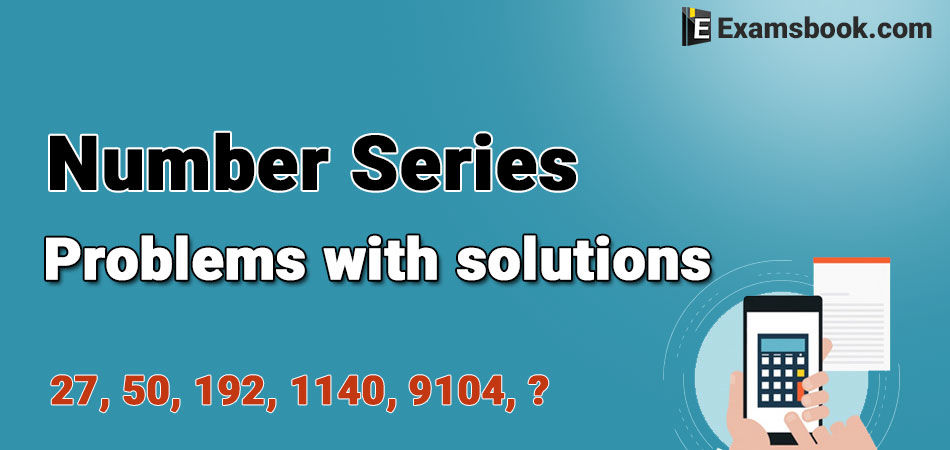• Save

# Number Series Problems with Solutions for SSC and Bank ExamsNumber series is an important topic to be asked in the competitive examination. A series is given in this topic, this series is based on a particular pattern of which a number is missing. Which we have to find out. These questions are a little hard but you can easily solve these with the pattern/solutions given here.

Preparation is the foundation of all competitive exams but it will be easy if you will do preparation with selective questions and answers. So, you can also practice Alphabet series questions and answers by one click.

As well as you should know Numbers Problems with Solutions to increase your performance in competitive exams.

## Number Series Problems with Solutions for Competitive Exams

Q.1. 2, 4, 10, 22, 42, 72, ?

(A) 102

(B) 106

(C) 114

(D) 124

(E) 132

Solution:

2+12 +1= 4

4+22 +2= 10

10+32 +3= 22

22+42 +4= 42

42+52 +5= 72

72+62+6 = 114

Q.2. 4, 2, 2, 4, 16, ?

(A) 64

(B) 72

(C) 96

(D) 128

(E) 156

Solution:

4* .5 = 2

2*1 = 2

2*2 = 4

4*4 = 16

16*8 = 128

Q.3. 15, 15, 30, 10, 40, ?, 48

(A) 8

(B) 20

(C) 24

(D) 40

(E) 60

Solution:

15/1 = 15

15*2 = 30

30/3 = 10

10*4 = 40

40/5 = 8

8*6 = 48

Q.4. 2, 3, 8, 27, 112, ?

(A) 156

(B) 224

(C) 375

(D) 480

(E) 565

Solution:

2*1+1 = 3

3*2+2 = 8

8*3+3 = 27

27*4+4 = 112

112*5+5 = 565

Q.5. 5, 6, 10, 33, 128, ?

(A) 375

(B) 445

(C) 565

(D) 645

(E) 675

Solution:

5*1+1 = 6

6*2-2 = 10

10*3+3 = 33

33*4-4 = 128

128*5+5 = 645

Q.6. 27, 50, 192, 1140, 9104, ?

(A) 90400

(B) 91020

(C) 92040

(D) 94060

(E) None of these

Solution:

27, 50, 192, 1140, 9104, ?

27*2-4 = 50

50*4-8 = 192

192*6-12 = 1140

1140*8-16 = 9104

9104*10-20 = 91020

Q.7. 6, 11, 32, 111, 464, ?

(A) 2345

(B) 2475

(C) 2525

(D) 3050

(E) None of these

Solution:

6, 11, 32, 111, 464, ?

6*1+5 = 11

11*2+10 = 32

32*3+15 = 111

111*4+20 = 464

464*5+25 = 2345

Q.8. 2, 12, 36, 80, ?, 252, 392

(A) 80

(B) 100

(C) 120

(D) 150

(E) None of these

Solution:

2, 12, 36, 80, ?, 252, 392

12+13 = 2

22+23 = 12

32+33 = 36

42+43 = 80

52+53 = 150

62+63 = 252

72+73 = 392

If have any doubt or want to ask anything related number series problems, feel free and ask me in the comment section. Visit on the next page to practice more number series problems with solutions.